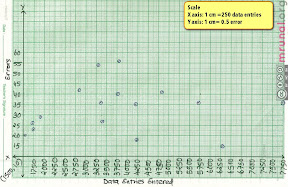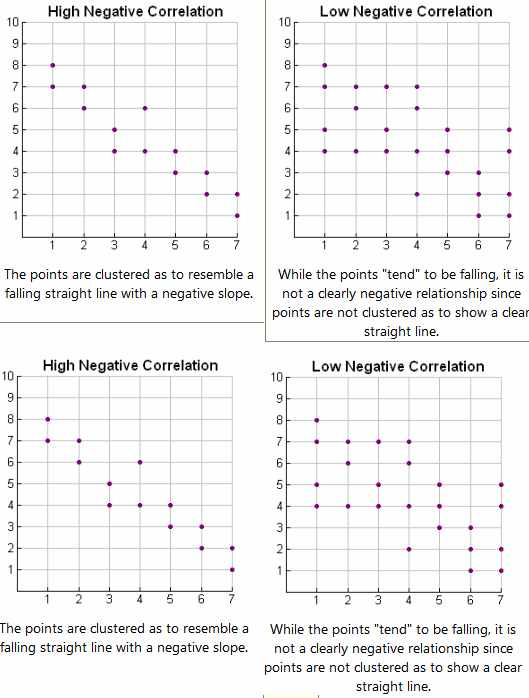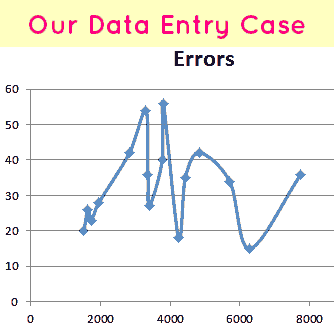# [Statistics 4GS] Scatter-plot Graph Question from GS (Mains) 2012: Solution, Explanation, Sample Graph

In Aptitude by Support Staff

This was asked in General Studies (Mains) 2012, Paper II of UPSC Civil Service Exam

The following table gives the number of mistakes made by 16 data entry clerks who enter medical data from case report forms. The column Entered indicates the number of values entered, and the column Errors gives the number of coding errors that were detected among these.

 Entered Errors 4434 35 4841 42 6280 15 1958 28 7749 36 2829 42 4239 18 3303 54 5706 34 3770 40 3363 36 1740 23 3404 27 1640 26 3803 56 1529 20

## Response Variable vs explanatory variable?

Q1. Make a scatter-plot of these data. Which do you choose for the response and which for the explanatory variables? Describe any patterns.

First, what is response variable and what is explanatory variable?

 Term Meaning Where to plot? X or Y? response variable Dependent variable) measures an outcome of a study.Also known as “Dependent Variable” Y explanatory variable explains or causes changes in the response variable. X
• Since the purpose of the given study/experiment is (/seems) to explore the effect of heavy workload on number of typing errors,
• therefore  the explanatory variable is No. of Entries Entered (X-axis),
• and the response variable is No. of errors Made (Y-axis).

Now time to make the scatter plot graph.

Second (and the most important) task is getting the right “SCALE” on x and y axis.

Let’s start with the easy one: Y axis

## Scale on Y axis

Take the difference between highest and lowest observation (56-15=41)

We want to take scales which are multiples of 2, 5 or 10. It makes easy to plot numbers. In this case, if we take 1cm =5 errors, then to plot 41 errors, we’d need 41/5=8+ cms. This can be accommodated easily.

So on Y axis, scale : 1 cm = 5 errors.

And in one cm there are 10mm. so 1 mm (the smallest sized box on graph)=5/10 errors= 0.5 errors. That means 2mm (set of two small sized boxes)=0.5+0.5=1 error.

## Scale on X axis

• Take the difference between highest and lowest observation (7749-1529)=6220.
• A normal graph paper is about 26cm x 20cm
• But we need to cutdown 1 cm on each side to label the axis.
• So practically we’re left with only 25×19 cm.
• Now obviously very hard to plot 6220 on the shorter side of graph paper (19cm side).
• Better construct the graph as ‘landscape layout’. i.e. we’ll take longer side (25cm) as our x-axis.
• If we take 1cm =200 entries,
• Then to plot 6220 entries, we’d need 6220/200= 31+ cm. =not possible, graph can give max 26cm.
• Go even bigger
• How about 1cm=250 entries?
• In that case, to plot 6220 entries, we’d need 6220/250=24.88 = very close but sufficient.
• Ok, so on X axis, Scale: 1cm = 250 entries.
• But then how to plot numbers?
• 1cm = 250 entries, so
• 1mm (smallest greenbox)=250/10=25 entries.
• So on x axis, 10 green boxes (1cm) will go on like this:
 cm 1 1.1 1.2 1.3 1.4 1.5 1.6 1.7 1.8 1.9 2 value(no. of Entries) 1500 1525 1550 1575 1600 1625(MIDPOINT) 1650 1675 1700 1725 1750

Question: how to plot the (1529, 20)

Well 20 (Y axis), is very easy because the scale is big enough.

But to get 1529, mark it between 1525 and 1550. Where exactly between 1525-1550? well, no one can know! The damn graph paper is so small. Anyways no need to worry, because we have to find correlation only and no median, so 100% accuracy while plotting the data, is not necessary.

Here is the sample answer: scatter plot graph, click to enlarge(^for illustration purpose only, this too may be containing some errors.)

## Correlation between two variables?

Q2. Does the scatter-plot indicate any relation between the two variables?

If there is any relation, it’d look like one of these graphs (images taken regentsprep.org)Our data entry graph shows no correlation because it doesn’t look like any of above four graphs.

If its still not clicking your mind, check out this excel graph of given data.Clearly the errors are going up and down, but without any pattern.

Ans. The Scatter plot does not indicate any relation between two variables (no. of entries and no. of errors)

## More work=More errors?

Q3. One analyst concluded,”The clerks who enter more values make more mistakes. Evidently they become tired as they enter more values.” Is this an appropriate conclusion?

This is analysis is flawed because of two reasons

• Reason 1. There is no correlation between No. Entries and No. of Errors, as we observed in the graph and Q2.
• Reason 2.  Let’s divide work as low-workload, medium workload, high workload, according to number of entries.
 Segments Low workload Medium High No. of Entries 1500-3600 3601-5700 5701-7800 No of points. 8 5 3 No of errors approx. 280 180 85

So, the clerks with heavy workload (more entries) are actually making less mistakes or errors compared to low-workload clerks.